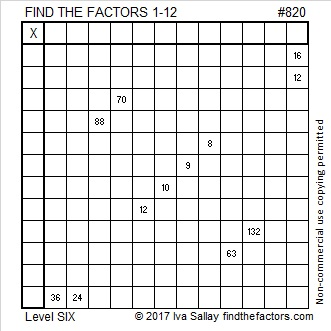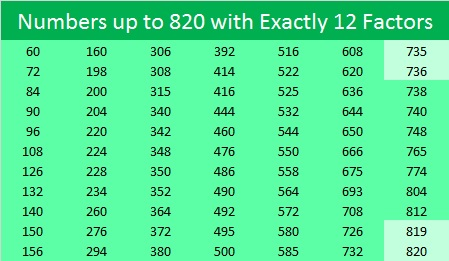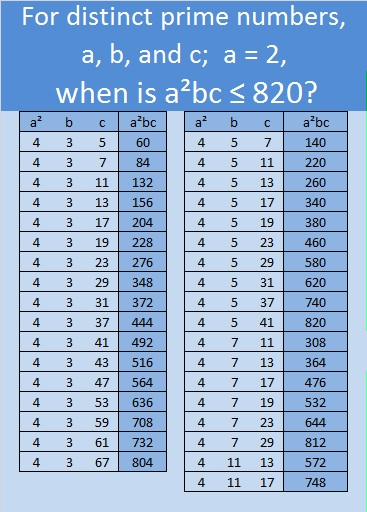# Numbers up to 820 with Exactly 12 Factors

Let’s begin with today’s puzzle. Afterwards I’ll tell you a little about the number 820 and why I decided to make a list of all the numbers up to 820 with exactly 12 factors.Print the puzzles or type the solution on this excel file: 12 factors 815-820

Now here’s some information about the number 820:

• 820 is a composite number.
• Prime factorization: 820 = 2 × 2 × 5 × 41, which can be written 820 = 2² × 5 × 41
• The exponents in the prime factorization are 2, 1, and 1. Adding one to each and multiplying we get (2 + 1)(1 + 1)(1 + 1) = 3 x 2 x 2 = 12. Therefore 820 has exactly 12 factors.
• Factors of 820: 1, 2, 4, 5, 10, 20, 41, 82, 164, 205, 410, 820
• Factor pairs: 820 = 1 × 820, 2 × 410, 4 × 205, 5 × 164, 10 × 82, or 20 × 41
• Taking the factor pair with the largest square number factor, we get √820 = (√4)(√205) = 2√205 ≈ 28.635642820 is the sum of two squares two ways:

• 28² + 6² = 820
• 26² + 12² = 820

Because 5 and 41 are two of its prime factors, 820 is the hypotenuse of FOUR Pythagorean triples:

• 180-800-820 which is 20 times 9-40-41
• 336-748-820 which is 4 times 84-187-205, but it could also be calculated from 2(28)(6), 28² – 6², 28² + 6²
• 492-656-820 which is 164 times 3-4-5
• 532-624-820 which is 4 times 133-156-205, but it could also be calculated from 26² – 12², 2(26)(12),26² + 12²

Since 820 = (40×41)/2, we know that 820 is the 40th triangular number, thus

• 1 + 2 + 3 + . . . + 38 + 39 + 40 = 820

820 is also a palindrome in four other bases:

• 1010101 BASE 3 because 3⁶+3⁴+3²+3⁰=820
• 1111 BASE 9 because 9³+9²+9¹+9⁰=820
• 868 BASE 11 because 8(11²)+6(11¹)+8(11⁰)=820
• 1I1 BASE 21 (I is 18 base 10) because 21²+18(21¹)+21⁰=820

Below is a chart of the numbers up to 820 with exactly 12 factors. Notice that two sets of consecutive numbers, (735, 736) and (819, 820), are on the list. Look at their prime factorizations:

• 735=3×5×7², 736=2⁵×23
• 819=3²×7×13, 820=2²×5×41Those prime factorizations mean that while 735 and 736 are the smallest consecutive numbers with exactly 12 factors, 819 and 820 are the smallest consecutive numbers whose prime factorizations consist of one prime number squared and exactly two other primes. Thanks to OEIS.org for alerting me to that fact. Here’s something interesting about the chart: of the 77 numbers listed, only six are odd numbers.

So, how did I know what numbers to put on the list?

In order to determine how many numbers up to 820 have exactly 12 factors, we must first factor 12. We know that 12=12, 6×2, 4×3, and 3×2×2.

Next we subtract 1 from each of those factors to determine the exponents we need to use:

12 gives us 12-1=11. For prime number a, when is a¹¹ not larger than 820? Never, because 2¹¹>820.

6×2 gives us 6-1=5 and 2-1=1. For prime numbers a and b, with a≠b, when is ab¹ less than or equal to 820? These nine times:

• 2×3=96, 2×5=160, 2×7=224, 2×11=352,
• 2×13=416, 2×17=544, 2×19=608, 2×23=736
• 3×2=486

4×3 gives us 4-1=3 and 3-1=2. For prime numbers a and b, with a≠b, when is a³b² not larger than 820? These six times:

• 2³×3²=72, 2³×5²=200, 2³×7²=392
• 3³×2²=108, 3³×5²=675
• 5³×2²=500

3×2×2 gives us 3-1=2, 2-1=1, and 2-1=1. For distinct prime numbers a, b, and c, when is a²bc not larger than 820? 52 times. Here’s the breakdown: It happens 35 times when 2²=4 is the square number:And it happens another 27 times when a prime number other than 2 is squared:That’s a lot of numbers with exactly 12 factors! After I sorted all the numbers that I found in numerical order, I was able to make that chart of numbers up to 820 with exactly 12 factors, and yes 819 and 820 are the smallest two consecutive numbers whose prime factorization consists exactly of one prime number squared and two other prime numbers.This site uses Akismet to reduce spam. Learn how your comment data is processed.